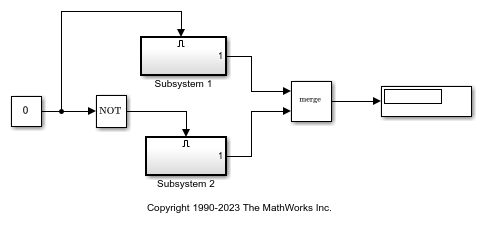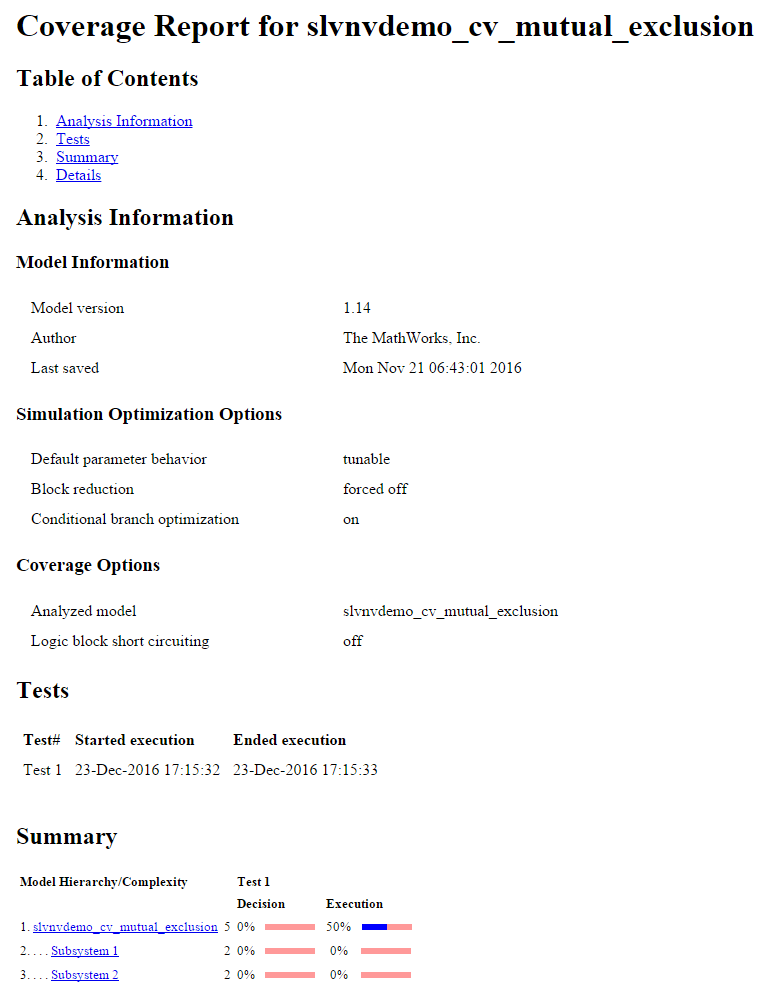Main Content

# Operations on Coverage Data

This example shows how to use the overloaded operators +, *, and - to combine coverage results into a union, intersection, or set difference of results.

### Example Model

Open a simple model with two mutually-exclusive enabled subsystems.

```open_system('slvnvdemo_cv_mutual_exclusion') ```Use the commands `cvtest` and `cvsim` to start simulation. Initially, the value of the Constant block is 0, which forces Subsystem 2 to execute.

```test1 = cvtest('slvnvdemo_cv_mutual_exclusion'); data1 = cvsim(test1) ```
```data1 = ... cvdata version: (R2021a) id: 231 type: TEST_DATA test: cvtest object rootID: 233 checksum: [1x1 struct] modelinfo: [1x1 struct] startTime: 23-Feb-2021 16:43:06 stopTime: 23-Feb-2021 16:43:06 intervalStartTime: 0 intervalStopTime: 0 simulationStartTime: 0 simulationStopTime: 10 filter: simMode: Normal ```

The following commands change the value of the Constant block to 1 before running the second simulation. This forces Subsystem 1 to execute.

```set_param('slvnvdemo_cv_mutual_exclusion/Constant','Value','1'); test2 = cvtest('slvnvdemo_cv_mutual_exclusion'); data2 = cvsim(test2) ```
```data2 = ... cvdata version: (R2021a) id: 286 type: TEST_DATA test: cvtest object rootID: 233 checksum: [1x1 struct] modelinfo: [1x1 struct] startTime: 23-Feb-2021 16:43:08 stopTime: 23-Feb-2021 16:43:08 intervalStartTime: 0 intervalStopTime: 0 simulationStartTime: 0 simulationStopTime: 10 filter: simMode: Normal ```

We use the `decisioninfo` command to extract the decision coverage from each test and list it as a percentage.

Note: While both tests have 50% decision coverage, whether or not they cover the same 50% is unknown.

```cov1 = decisioninfo(data1,'slvnvdemo_cv_mutual_exclusion'); percent1 = 100*(cov1(1)/cov1(2)) cov2 = decisioninfo(data2,'slvnvdemo_cv_mutual_exclusion'); percent2 = 100*(cov2(1)/cov2(2)) ```
```percent1 = 50 percent2 = 50 ```

### Finding the Union of Coverage

Use the + operator to derive a third cvdata object representing the union of data1 and data2 cvdata objects.

Note: New cvdata objects created from combinations of other simulation results are marked with the type property set as DERIVED_DATA.

```dataUnion = data1 + data2 ```
```dataUnion = ... cvdata version: (R2021a) id: 0 type: DERIVED_DATA test: [] rootID: 233 checksum: [1x1 struct] modelinfo: [1x1 struct] startTime: 23-Feb-2021 16:43:06 stopTime: 23-Feb-2021 16:43:08 intervalStartTime: 0 intervalStopTime: 0 filter: simMode: Normal ```

Notice that the union of the coverage is 100% because there is no overlap in the coverage between the two sets.

```covU = decisioninfo(dataUnion,'slvnvdemo_cv_mutual_exclusion'); percentU = 100*(covU(1)/covU(2)) ```
```percentU = 100 ```

### Finding the Intersection of Coverage

Confirm that the coverage does not overlap between the two tests by intersecting data1 and data2 with the * operator. As expected, there is 0% decision coverage in the intersection.

```dataIntersection = data1 * data2 covI = decisioninfo(dataIntersection,'slvnvdemo_cv_mutual_exclusion'); percentI = 100*(covI(1)/covI(2)) ```
```dataIntersection = ... cvdata version: (R2021a) id: 0 type: DERIVED_DATA test: [] rootID: 233 checksum: [1x1 struct] modelinfo: [1x1 struct] startTime: 23-Feb-2021 16:43:06 stopTime: 23-Feb-2021 16:43:08 intervalStartTime: 0 intervalStopTime: 0 filter: simMode: Normal percentI = 0 ```

### Using Derived Coverage Data Objects

Derived cvdata objects can be used in all reporting and analysis commands, and as inputs to subsequent operations. As an example, generate a coverage report from the derived dataIntersection object.

```cvhtml('intersect_cov', dataIntersection); % Input to another operation newUnion = dataUnion + dataIntersection ```
```newUnion = ... cvdata version: (R2021a) id: 0 type: DERIVED_DATA test: [] rootID: 233 checksum: [1x1 struct] modelinfo: [1x1 struct] startTime: 23-Feb-2021 16:43:06 stopTime: 23-Feb-2021 16:43:08 intervalStartTime: 0 intervalStopTime: 0 filter: simMode: Normal ```### Computing the Coverage (set) Difference

The - operator is used to form a cvdata object that represents the set difference between left and right operands. The result of the operation contains the coverage points that are satisfied in the left operand but not satisfied in the right operand. This operation is useful for determining how much additional coverage is attributed to a particular test.

In the following example, the difference between the union of the first and second test coverage and the first test coverage should indicate how much additional coverage the second test provided. As already shown, since none of the decision coverage points overlapped, the new decision coverage from test 2 is 50%.

```newCov2 = dataUnion - data1 covN = decisioninfo(newCov2,'slvnvdemo_cv_mutual_exclusion'); percentN = 100*(covN(1)/covN(2)) ```
```newCov2 = ... cvdata version: (R2021a) id: 0 type: DERIVED_DATA test: [] rootID: 233 checksum: [1x1 struct] modelinfo: [1x1 struct] startTime: 23-Feb-2021 16:43:06 stopTime: 23-Feb-2021 16:43:08 intervalStartTime: 0 intervalStopTime: 0 filter: simMode: Normal percentN = 50 ```

Get resources